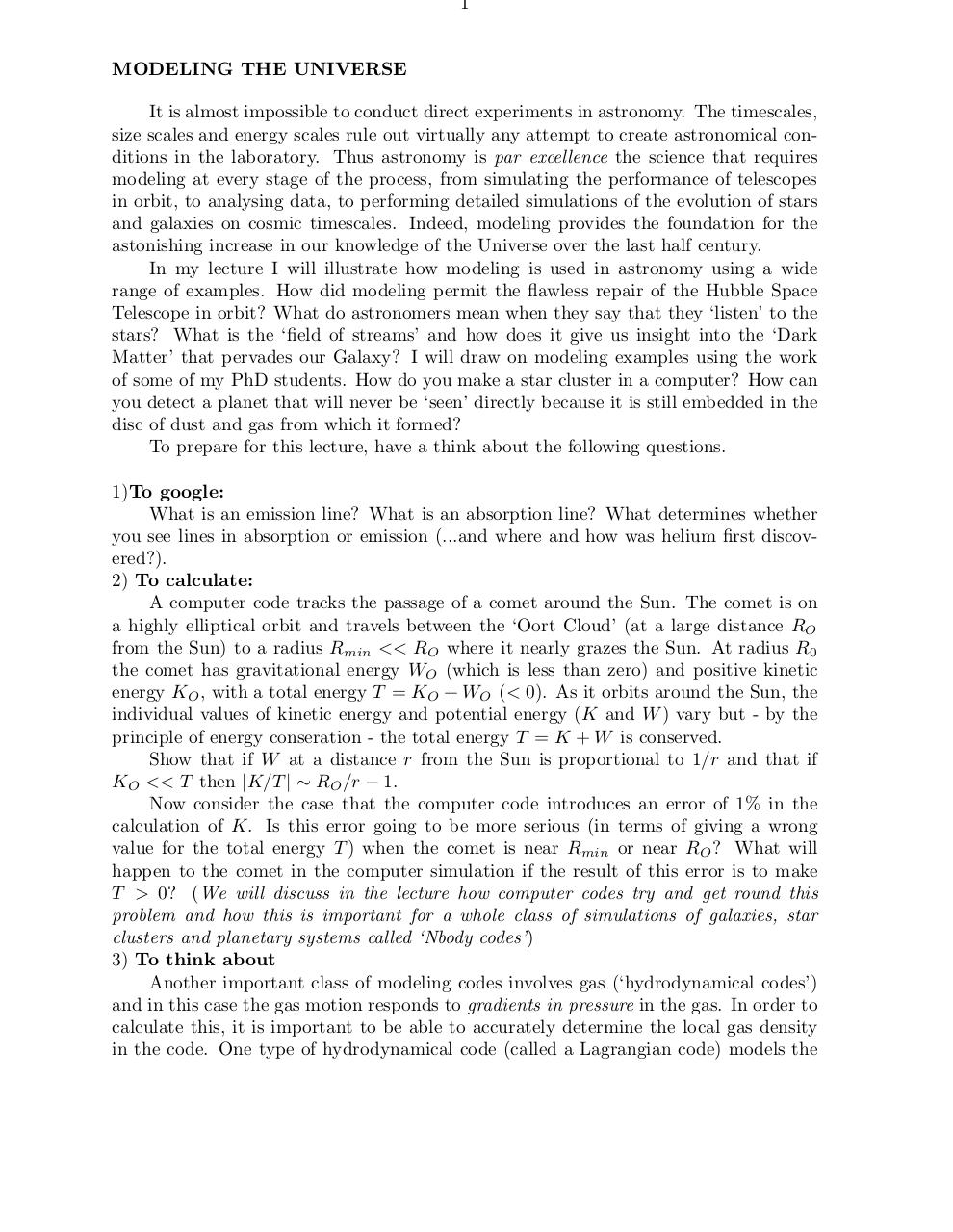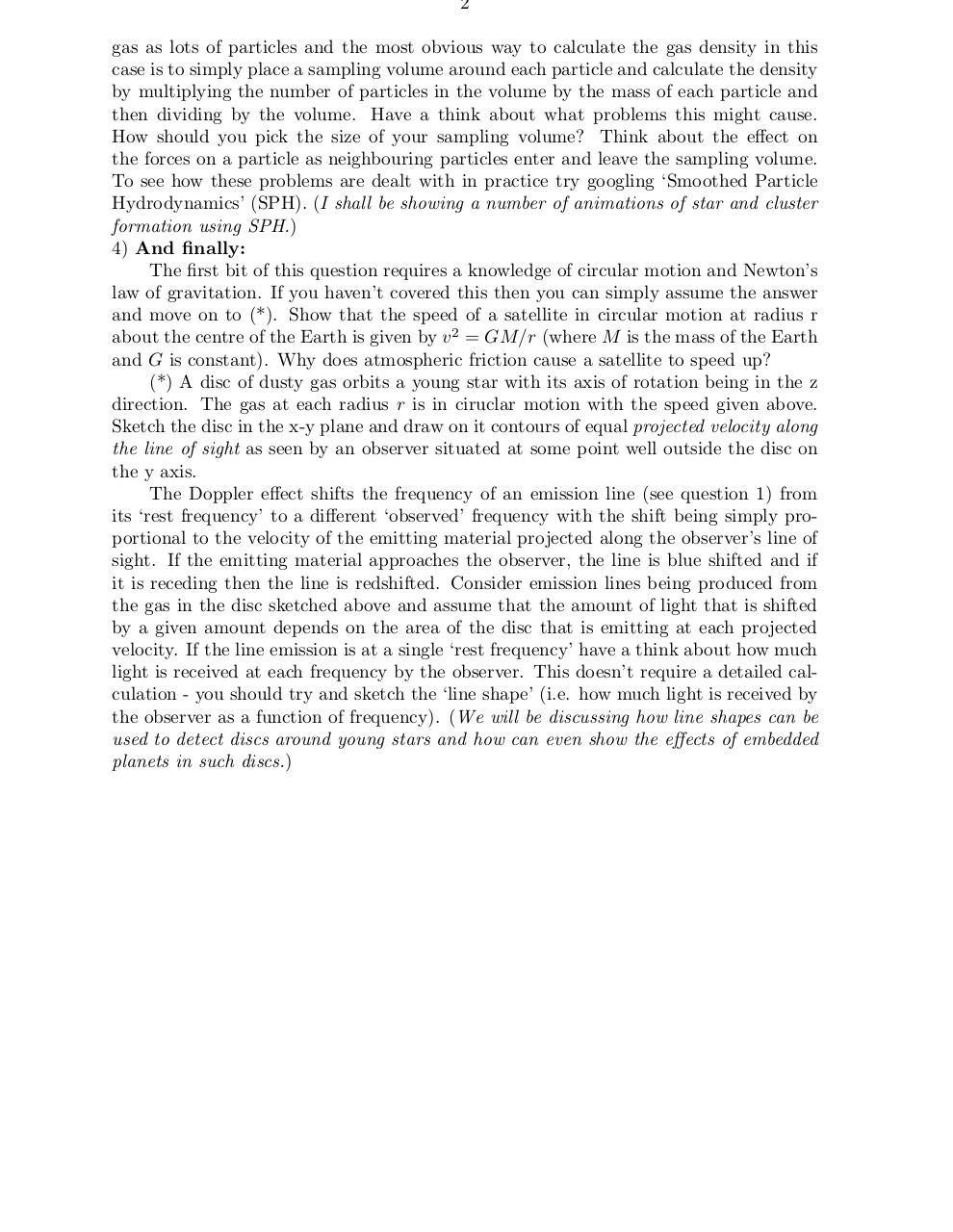# 1 Modelling the Universe .pdf

### File information

Original filename: 1 - Modelling the Universe.pdf
Title: stem.dvi

This PDF 1.4 document has been generated by dvips(k) 5.92b Copyright 2002 Radical Eye Software / GPL Ghostscript 9.0, and has been sent on pdf-archive.com on 05/02/2014 at 11:52, from IP address 194.81.x.x. The current document download page has been viewed 657 times.
File size: 40 KB (2 pages).
Privacy: public file

1 - Modelling the Universe.pdf (PDF, 40 KB)

### Document preview

1

MODELING THE UNIVERSE
It is almost impossible to conduct direct experiments in astronomy. The timescales,
size scales and energy scales rule out virtually any attempt to create astronomical conditions in the laboratory. Thus astronomy is par excellence the science that requires
modeling at every stage of the process, from simulating the performance of telescopes
in orbit, to analysing data, to performing detailed simulations of the evolution of stars
and galaxies on cosmic timescales. Indeed, modeling provides the foundation for the
astonishing increase in our knowledge of the Universe over the last half century.
In my lecture I will illustrate how modeling is used in astronomy using a wide
range of examples. How did modeling permit the flawless repair of the Hubble Space
Telescope in orbit? What do astronomers mean when they say that they ‘listen’ to the
stars? What is the ‘field of streams’ and how does it give us insight into the ‘Dark
Matter’ that pervades our Galaxy? I will draw on modeling examples using the work
of some of my PhD students. How do you make a star cluster in a computer? How can
you detect a planet that will never be ‘seen’ directly because it is still embedded in the
disc of dust and gas from which it formed?
To prepare for this lecture, have a think about the following questions.
What is an emission line? What is an absorption line? What determines whether
you see lines in absorption or emission (...and where and how was helium first discovered?).
2) To calculate:
A computer code tracks the passage of a comet around the Sun. The comet is on
a highly elliptical orbit and travels between the ‘Oort Cloud’ (at a large distance RO
from the Sun) to a radius Rmin &lt;&lt; RO where it nearly grazes the Sun. At radius R0
the comet has gravitational energy WO (which is less than zero) and positive kinetic
energy KO , with a total energy T = KO + WO (&lt; 0). As it orbits around the Sun, the
individual values of kinetic energy and potential energy (K and W ) vary but - by the
principle of energy conseration - the total energy T = K + W is conserved.
Show that if W at a distance r from the Sun is proportional to 1/r and that if
KO &lt;&lt; T then |K/T | ∼ RO /r − 1.
Now consider the case that the computer code introduces an error of 1% in the
calculation of K. Is this error going to be more serious (in terms of giving a wrong
value for the total energy T ) when the comet is near Rmin or near RO ? What will
happen to the comet in the computer simulation if the result of this error is to make
T &gt; 0? (We will discuss in the lecture how computer codes try and get round this
problem and how this is important for a whole class of simulations of galaxies, star
clusters and planetary systems called ‘Nbody codes’)
Another important class of modeling codes involves gas (‘hydrodynamical codes’)
and in this case the gas motion responds to gradients in pressure in the gas. In order to
calculate this, it is important to be able to accurately determine the local gas density
in the code. One type of hydrodynamical code (called a Lagrangian code) models the

2
gas as lots of particles and the most obvious way to calculate the gas density in this
case is to simply place a sampling volume around each particle and calculate the density
by multiplying the number of particles in the volume by the mass of each particle and
then dividing by the volume. Have a think about what problems this might cause.
How should you pick the size of your sampling volume? Think about the effect on
the forces on a particle as neighbouring particles enter and leave the sampling volume.
To see how these problems are dealt with in practice try googling ‘Smoothed Particle
Hydrodynamics’ (SPH). (I shall be showing a number of animations of star and cluster
formation using SPH.)
4) And finally:
The first bit of this question requires a knowledge of circular motion and Newton’s
law of gravitation. If you haven’t covered this then you can simply assume the answer
and move on to (*). Show that the speed of a satellite in circular motion at radius r
about the centre of the Earth is given by v 2 = GM/r (where M is the mass of the Earth
and G is constant). Why does atmospheric friction cause a satellite to speed up?
(*) A disc of dusty gas orbits a young star with its axis of rotation being in the z
direction. The gas at each radius r is in ciruclar motion with the speed given above.
Sketch the disc in the x-y plane and draw on it contours of equal projected velocity along
the line of sight as seen by an observer situated at some point well outside the disc on
the y axis.
The Doppler effect shifts the frequency of an emission line (see question 1) from
its ‘rest frequency’ to a different ‘observed’ frequency with the shift being simply proportional to the velocity of the emitting material projected along the observer’s line of
sight. If the emitting material approaches the observer, the line is blue shifted and if
it is receding then the line is redshifted. Consider emission lines being produced from
the gas in the disc sketched above and assume that the amount of light that is shifted
by a given amount depends on the area of the disc that is emitting at each projected
velocity. If the line emission is at a single ‘rest frequency’ have a think about how much
light is received at each frequency by the observer. This doesn’t require a detailed calculation - you should try and sketch the ‘line shape’ (i.e. how much light is received by
the observer as a function of frequency). (We will be discussing how line shapes can be
used to detect discs around young stars and how can even show the effects of embedded
planets in such discs.)#### HTML Code

Copy the following HTML code to share your document on a Website or Blog

#### QR Code### Related keywords